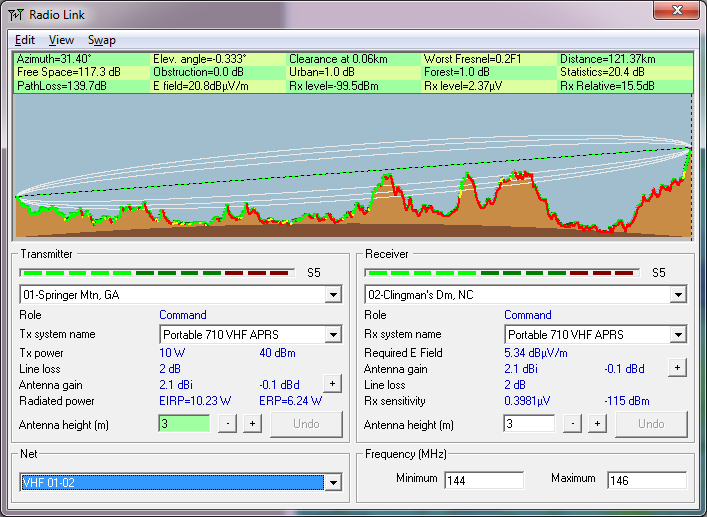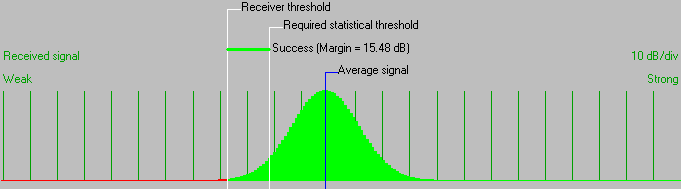• Distance between 01-Springer Mtn, GA and 02-Clingman's Dm, NC is 121.4 km (75.4 miles)
• True North Azimuth = 31.40°, Magnetic North Azimuth = 36.40°, Elevation angle = -0.3329°
• Terrain elevation variation is 1418.7 m
• Propagation mode is line-of-sight, minimum clearance 0.2F1 at 0.1km
• Average frequency is 145.000 MHz
• Free Space = 117.3 dB, Obstruction = 0.0 dB, Urban = 1.0 dB, Forest = 1.0 dB, Statistics = 20.4 dB
• Total propagation loss is 139.7 dB (interference mode (optimistic))
• System gain from 01-Springer Mtn, GA to 02-Clingman's Dm, NC is 155.2 dB
• System gain from 02-Clingman's Dm, NC to 01-Springer Mtn, GA is 155.2 dB
• Worst reception is 15.5 dB over the required signal to meet 95.000% of situations